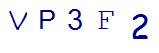# Third-Grade Math Help to Conquer DivisionLast Updated on August 31, 2021 by Thinkster

Of the four basic math operations that early grade-schoolers learn, division is usually taught last, after the foundations of addition, subtraction, and multiplication have been laid. This order makes logical sense, but it also can create a perception in third-graders’ minds that division is the hardest to learn. You get nice, clean answers with addition and multiplication. But division comes with remainders, and the dividend and divisor have to be in the right place or else everything goes haywire, and what’s with that goofy squiggly used for long division? All this can make your child require third-grade math help with division.

Luckily, multiplication and division are complementary operations, so many of the strategies kids use to multiply can be adapted to division. If your child is in need of third-grade math help, here are some ways to help them divide and conquer:

Divide Objects into Groups

This activity provides a good visualization of the division process. First, assemble a large group of objects. They can be anything—pennies, Legos, Pokemon cards—as long as there is enough to easily divide. Then, direct your child to create a certain number of equal groups. For example, if you have 60 pretzels, tell him to see how many groups of 10 he can make. Start out with numbers that won’t result in any objects being left over, but eventually, be tricky, by borrowing from the last example, seeing how many groups of 7 he can form from 60 pretzels. Besides increasing the challenge, this is a good introduction to remainders.

Skip Counting

Usually used to help with multiplication, skip counting can also be effective for third-grade math division. Instead of skip counting a certain number of times and discovering the answer, your child can skip count to a larger number and keep track of how many times she added. For example, 30÷6 would be skip counted 6-12-18-24-30 to give an answer of 5.

Repeated Subtraction

Repeated subtraction is essentially skip counting in reverse—you start from a larger dividend and subtract backward from the divisor. So, if the equation was 42÷7, subtract by 7 until you get to 0: 35-28-21-14-7-0, to get an answer of 6.

Draw It!

Much third-grade math help relies on visualization to paint a clear picture of the problem at hand. Students learning how to divide can always illustrate the equation to arrive at the answer. For example, if the problem is 24÷6, your child could draw rows of 6 x’s (or stars, or tally marks, or whatever helps him or her learn best) until they reach 24, and then count up the rows for the answer. Similar to dividing objects, this also can provide a good introduction to remainders.

What third-grade math help has been beneficial for your child while learning division?

SummaryArticle Name
Third-Grade Math Help to Conquer Division
Description
Division is usually the most challenging of the four basic math concepts. Here are some ways you can provide third-grade math help to your child.
Author
Publisher Name
Thinkster Math
Publisher Logo

## Subscribe to Thinkster Blog## Recommended Articles### Mastering Math: How to Crack Challenging Math Problems in Under a Minute!

Math, often regarded as the king of all sciences, can sometimes pose challenges that leave even the brighte...